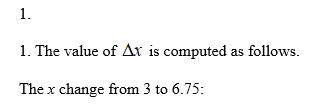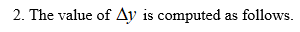# Suppose the value of yy and the value of xx vary together at a constant rate of change (so that Δy=0.75⋅Δx), and y=1.5 when x=3.Suppose the value of xx varies from x=3 to x=6.75.How much did the value of x change?Δx=How much did the value of y change over this interval of x?Δy=  What is the value of y when x=6.75?

Question
37 views

Suppose the value of yy and the value of xx vary together at a constant rate of change (so that Δy=0.75⋅Δx), and y=1.5 when x=3.

1. Suppose the value of xx varies from x=3 to x=6.75.

1. How much did the value of x change?

Δx=

2. How much did the value of y change over this interval of x?

Δy=

2. What is the value of y when x=6.75?

check_circle

Step 1Step 2...

### Want to see the full answer?

See Solution

#### Want to see this answer and more?

Solutions are written by subject experts who are available 24/7. Questions are typically answered within 1 hour.*

See Solution
*Response times may vary by subject and question.
Tagged in

### Other